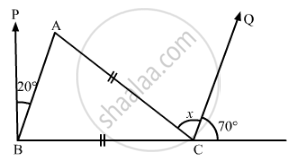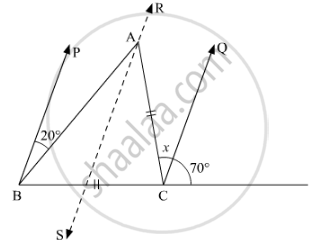Advertisement Remove all ads

# In the Given Figure, If Bp || Cq and Ac = Bc, Then the Measure of X is - Mathematics

MCQ

In the given figure, if BP || CQ and AC = BC, then the measure of x is#### Options

•  20°

• 25°

•  30°

• 35°

Advertisement Remove all ads

#### Solution

In the given figure,  BP || CQ and  AC || BC

We need to find the measure of xHere, we draw a line RS parallel to BP, i.e BP || RS

Also, using the property, “two lines parallel to the same line are parallel to each other”

As,

BP || RS

BP || CQ

Thus, RS || CQ

Now,  BP || RS  and BA is the transversal, so using the property, “alternate interior angles are equal”

∠PBA = ∠BAS

∠BAS = 20°          .......... (1)

Similarly,  CQ|| RS and AC is the transversal

∠QCA = ∠SAC

∠SAC = x                              ........(2)

Adding (1) and (2), we get

∠SAC + ∠BAS = 20° + x

∠A = 20° + x

Also, as  AC = BC

Using the property,”angles opposite to equal sides are equal”, we get

∠ABC = ∠CAB

∠ABC = 20° + x

Further, using the property, “an exterior angle is equal to the sum of the two opposite interior angles”

In ΔABC

ext. ∠C = ∠CAB + ∠ABC

70° + x = 20° + x + 20° + x

70° + x = 40° + 2x

70° - 40° = 2x - x

x = 30°

Thus,  x = 30°

Is there an error in this question or solution?
Advertisement Remove all ads

#### APPEARS IN

RD Sharma Mathematics for Class 9
Chapter 11 Triangle and its Angles
Q 22 | Page 28
Advertisement Remove all ads

#### Video TutorialsVIEW ALL 

Advertisement Remove all ads
Share
Notifications

View all notifications

Forgot password?
Course